# Tiler

On what surface m2 tiler must lay 30 square tiles, each of which has a side 25 cm?

S =  1.875 m2

### Step-by-step explanation: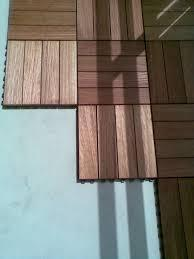Did you find an error or inaccuracy? Feel free to write us. Thank you!Tips to related online calculators
Do you want to convert length units?

#### You need to know the following knowledge to solve this word math problem:

We encourage you to watch this tutorial video on this math problem:

## Related math problems and questions:

• Tiles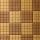How many square tiles with an area of 121 cm2 have to be ordered for the square room's paving with a side length of 2.75 meters?
• TilesHow many tiles of 20 cm and 30 cm can build a square if we have a maximum of 100 tiles?
• Cloth / textileWe have cloth measure 16 square meters. How many 20 cm by 20 cm by 8 cm bags you can make? Assume bag is a cuboid without one top base.
• Pool tilesThe pool is 25m long, 10m wide, and 160cm deep. How many m2 of tiles will be needed on the walls and the pool? How many tiles are needed when 1 tile has a square shape with a 20cm side? How much does it cost when 1m2 of tiles costs 258 Kc?
• The corridor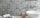The corridor is 12 m long and 3.6 m wide. It must be paved with rectangular tiles measuring 15 cm and 30 cm. Are 1000 pieces of tiles enough to pave the corridor?
• Pavilion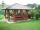The rectangular pavilion with dimensions 3.5 m and 2.75 m to be paved with square tiles of side 25 cm price of CZK 22 per 1 piece or rectangular tiles with sides of 20 cm and 15 cm in the price of CZK 11 per 1 pc. Which solution is cheaper (write its pric
• Flooring a roomFind the cost of flooring a room 6.5 m by 5 m with square tiles of sides 25 cm at the rate of rupees 9.40 per tile.
• HallRectangular hall will have pave by square tiles with a side length 15 cm. The hall has length 18 meters and width 3 m. How many tiles need to buy if 2 percent of the amount is disrupted during the work?
• Four prismsQuestion No. 1: The prism has the dimensions a = 2.5 cm, b = 100 mm, c = 12 cm. What is its volume? a) 3000 cm2 b) 300 cm2 c) 3000 cm3 d) 300 cm3 Question No.2: The prism base is a rhombus with a side length of 30 cm and a height of 27 cm. The height of t
• Empty aquariumHow much does an empty aquarium weigh with dimensions: length = 40 cm, width = 30 cm, height = 20 cm, if 1 dm2 of glass weighs 300 g? Calculate its weight in kilograms.
• RectanglesCalculate how many squares/rectangles of size 4×3 cm can be cut from a sheet of paper of 36 cm × 32 cm?
• TrevorTrevor wishes to tile the floor of his bathroom. The bathroom is rectangular and is 4.2m long and 3.3m wide. The tiles are 30cm by 30cm. Calculate the cost to tile the floor if each tile costs 72 cents.
• The bookThe book has 280 pages. Each side is a rectangle with sides 15 cm and 22 cm. a) how many leaves have the book? b) at least how many square meters of paper should be used to produce this book?
• Cuboid aquariumCuboid 25 times 30 cm. How long is third side if cuboid contains 30 liters of water?
• TilesFrom how many tiles 20 cm by 30 cm, we can build a square of maximum dimensions if we have maximum 881 tiles.
• CanopyMr Peter has a metal roof cone shape with a height of 127 cm and radius 130 cm over well. He needs to paint the roof with anticorrosion. How many kg of color must he buy if the manufacturer specifies the consumption of 1 kg to 3.3 m2?
• Star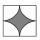Calculate the content of the shaded part of square with side 28 cm.